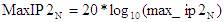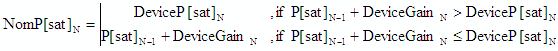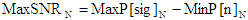Chapter 1, 2, 3, 4, 5, 6, 7, 8, 9, 10, 11, 12, 13

Version 1.11
by Kirt Blattenberger
RF Cafe Website
(www.rfcafe.com)

Chapter 5

5    Inband Output (Calculated) Parameters

Although the definitions of system cascaded parameters are pretty much standard throughout the industry, all of the formulas and assumptions used in RF Cascade Workbook are presented here in order to eliminate any ambiguity. Since formulas are contained in VBA code, you may easily modify them to suit your individual preferences without having to edit sometimes lengthy formulas directly within the cells.

One tip when viewing the cell formulas is to initially place the cursor in the cell, then click the “fx” icon on the tool bar. This will call up the function dialog box as shown in Figure 8 and make modification and evaluation of the function output simpler because the result will be displayed as parameters are changed.

span="3">- Nom -

 This section presents all of the formulas used in the VBA functions to calculate system cascade parameters. In the following equations, the subscript “N” refers to the current stage device parameter, while “N-1” refers to the cascaded system parameters up through and including the previous stage. Use Figure 7 as a reference when interpreting the formulas. For example, GainN refers to the gain of the current stage’s device. GainN-1 refers to the cascaded gain of all stages preceding the current stage. So referring to Figure 7, for N=3, DeviceGainN = -0.15 dB, and NomGainN-1 = -0.5 dB. Finally, upper case names refer to decibel values (e.g., G = 20 dB or P = 20 dBm), while lower case names refer to linear values (e.g., g = 100 or p = 100 mW). Min/max formulas are constructed using combinations most likely to occur based on the available combinations of parameters, rather than just those combinations that yield absolutely the largest and smallest values. For instance, the maximum IMD3 value uses the cascaded values of MaxP[sig] and MaxIP3 because both use MaxGain. You may easily modify the Min/Max formula variables to suit your particular needs.Figure 7    Formula Reference DesignationsFigure 8    Function (fx) Dialog Box Convert between decibels and non decibels as follows: P(dB) = 10*log10 (p)At the bottom of the calculated OIP2 and OIP3 columns, there is a row titled “Input Reference (dBm)” that contains the equivalent system input referenced values for the Nom, Min and Max cases.5.1    Gain (dB) Gain values expressed in decibels add arithmetically for the linear operating region (possible output compression is ignored). If the “Use VSWR” cell is set to “Y,” then the interstage VSWR mismatch values are added to the total gain for Min and Max values. Mismatch loss is not included in the calculation due to the complexity involved in making a full accounting of the effects – reverse isolation of preceding components, multiple reflection cycles and other phenomenon would have to be accommodated, and is therefore way beyond the scope of this workbook. - Nom - Nominal cascaded gain uses the sum of the nominal gains for each of the stages.- Max - Maximum cascaded gain uses the sum of the maximum gains of each of the stages.- Min - Minimum cascaded gain uses the sum of the minimum gains for each of the stages.5.2    Noise Figure, NF (dB) Noise figure is effectively the reduction of signal to noise ratio from the cascade input to the output. Noise figure must be calculated using the non decibel (linear) forms of gain and noise figure, and then converted into decibels. These equations deal with power, so the “10*log10 (v)” decibel form is used. Image Noise Image noise, which can and often does contribute to the total noise power in systems, is not accounted for in RF Cascade Workbook. Image noise is added to the system when frequency mixing is performed and the image frequency band at the input of the mixer is not sufficiently filtered. The image frequency is defined as 2*LO-1*RF. Unless the mixer is an image-reject model specifically designed to attenuate the image frequency, it will translate the image band content (even if it is only noise) into the IF band just as it will the intended signals. The result is increased noise power that, worst case, could add 3 dB to the noise floor. If your system can tolerate the hit in sensitivity, then there is no problem. If you must maintain the highest level of sensitivity, then consider placing a filter before the mixer that will know the image band content down sufficiently to be negligible at the mixer output. 10 dB of attenuation is a good minimum target for just thermal noise, but more could be required if there is also signal content in the image band. - Nom - Nominal cascaded noise figure uses the nominal gains and noise figures of each of the stages.- Max - Maximum cascaded noise figure uses the minimum gains and maximum noise figures of each of the stages.- Min - Minimum cascaded noise figure uses the maximum gains and minimum noise figures of each of the stages.5.3    2-Tone, 2nd-Order Intercept Point, OIP2 (dBm) IP2 is the theoretical power at which the 2nd-order intermodulation products would intersect the power of the original tones (CW) when input/output power slopes are plotted. In the linear region of operation, the original tones plot on a 1:1 (normalized) slope, while the 2nd-order products plot on a 2:1 (normalized) slope. Therefore, the product tones increase at twice the rate of the original tones, and the lines cross at the IP2 point. See Figure 9 for visualization. 2nd-order intermodulation products are an important consideration in direct conversion and near zero IF systems.Figure 9    Intercept Points & Saturated Power - Nom - Nominal cascaded 2nd-order intercept point uses the nominal gains and IP2s of each of the stages.- Max - Maximum cascaded 2nd-order intercept point uses the maximum gains and maximum IP2s of each of the stages.- Min - Minimum cascaded 2nd-order intercept point uses the minimum gains and minimum IP2s of each of the stages.5.4    2-Tone, 3rd-Order Intercept Point, IP3 (dBm) IP3 is the theoretical power at which the 3rd-order intermodulation products would intersect the power of the original tones (CW) when input/output power slopes are plotted. In the linear region of operation, the original tones plot on a 1:1 (normalized) slope, while the 3rd-order products plot on a 3:1 (normalized) slope. Therefore, the product tones increase at three times the rate of the original tones, and the lines cross at the IP3 point. See Figure 9 for visualization. - Nom - Nominal cascaded 3rd-order intercept point uses the nominal gains and IP3s of each of the stages.- Max - Maximum cascaded 3rd-order intercept point uses the maximum gains and maximum IP3s of each of the stages.- Min - Minimum cascaded 3rd-order intercept point uses the minimum gains and minimum IP3s of each of the stages.5.5    Saturated Power, P[sat] (dBm) P[sat] is the output power at which no further increase in the input power will result in an increase at the output. This, along with the 1 dB compression point (P1dB) is a very nonlinear region of operation and can only be modeled by sophisticated transfer functions that are unique to each component. See Figure 9 for visualization. Therefore, no attempt is made to model it here. Instead, the P[sat] value is used as a monitor for the power level in the system to alert the user to a potential problem. A check is made to determine whether the power level at the component input, plus the linear gain of the component, results in a power level equal to or greater than the P[sat] of the component. If so, then the output power is limited to the component’s P[sat] power level. No tolerance input parameter is provided for P[sat] because it normally is not an intentional design parameter. Note: The cascaded signal power level displayed in the worksheet is calculated as if there is no saturation limit. - Nom - Nominal gain and P[sat] values are used per the following equation.- Max - Not used. - Min - Not used. 5.6    Signal Power, P[sig] (dBm) P[sig] is the power of the signal as it propagates through the cascade, and is increased or decreased by the linear gain of each stage. Note that it is possible for the calculated value to exceed the P[sat] value, because no adjustment is made. This is done to prevent the annoying case where all of the other power dependant values are thrown off by an adjusted output power value. There is an indication of a saturated condition given in the component parameter input area column labeled “!!!.” - Nom -- Max -- Min -5.7    Noise Bandwidth, NBW (Freq Units) Cascaded noise bandwidth merely checks the NBW of the current component, and sets the system NBW to the lesser of either the component NBW or the system’s previous NBW. Only a nominal value is calculated. Frequency units are set on the “Filter-Mixer” worksheet. This value is used with calculations that include noise power levels, like dynamic range and spurious free dynamic range.- Max - Not used. - Min - Not used. 5.8    Noise Power, P[n] (dBm) P[n] is the power of the noise as it propagates through the cascade, and is increased or decreased by the gain, noise figure and NBW of each stage. Since the system temperature is given in Celsius degrees, 273.15 is added to get equivalent Kelvin degrees. NWB is given in whatever Frequency Units are specified on the “Filter-Mixer” worksheet, so a multiplication by the appropriate factor is done. Note that MaxGain is used with MaxNF and MinGain is used with MinNF. This is because in most systems the noise figure is set near the cascade input where the least amount of gain has accumulated. Your specific application might warrant a different combination of Min/Max values. See section 5.2 for discussion on image noise contribution to noise power. In the following equations, k is Boltzmann's constant. - Nom -- Max -- Min -, where:5.9    Signal to Noise Ratio, SNR (dB) SNR is the difference between the noise power level and the signal power level. - Nom -- Max -- Min -5.10    Saturated Dynamic Range, SDR (dB) SDR is the difference between the saturated power level and the noise power level, minus the minimum system SNR, as specified in the system parameter area (Min SNR). This is different than the traditional dynamic range (DR), which references the 1 dB compression point (P1dB); RF Cascade Workbook 2004 does not calculate P1dB. Since P[sat] is typically about 2 or 3 dB above P1dB, the traditional dynamic range will be about 2 or 3 dB lower. - Nom -- Max -- Min -5.11    2nd-Order Spurious-Free Dynamic Range, SFDR2 (dB) SFDR2 is the difference between the IP2 power and the theoretical power of two tones at the system input that would generate 2nd order products at the output with a power just equal to the noise power at the output. - Nom -- Max -- Min -5.12    2nd Order Intermodulation Product Power, IMD2 (dBm) Calculations of IMD2 in RF Cascade Workbook 2004 assume intermod products are caused by the nonlinear mixing of two input tones of equal amplitude. It is essentially the same process as in a mixer for frequency conversion, where an infinite series is produced that consists of every possible frequency according to ±j*Tone1 ±k*Tone2. 2nd order products are more likely to fall inband for the direct conversion system popular these days. A smaller (minimum) IMD2 is better.- Max -- Min -5.13    Delta 2nd Order Intermodulation Products, ΔIMD2 (dB) Delta IMD2 intermod is the difference between the IMD2 product power (IMD2) and the signal power, P[sig]. - Nom -- Max -- Min -5.14    3rd-Order Spurious-Free Dynamic Range, SFDR3 (dB) SFDR3 is the difference between the IP3 power and the theoretical power of two tones at the system input that would generate 3rd order products at the output with a power just equal to the noise power at the output. - Nom -- Max -- Min -5.15    3rd Order Intermodulation Product Power, IMD3 (dBm) Calculations of IMD3 in RF Cascade Workbook 2004 assume intermod products are caused by the nonlinear mixing of two input tones of equal amplitude. It is essentially the same process as in a mixer for frequency conversion, where an infinite series is produced that consists of every possible frequency according to ±j*Tone1 ±k*Tone2. The 3rd order products that most likely fall inband at the output are ±2*Tone1 ±Tone2 and ±Tone1 ±2*Tone2. In reality, the powers of most products are below the noise power. A smaller (minimum) IMD3 is better. - Nom -- Max -- Min -5.16    Delta 3rd Order Intermodulation Products, ΔIMD3 (dB) Delta IMD3 intermod is the difference between the IMD3 product power (IMD3) and the signal power, P[sig]. - Nom -- Max -- Min -5.17    Interstage VSWR Mismatch Error (dB) VSWR mismatch errors are caused by constructive and destructive interference of the voltage standing waves at component interfaces due to impedance mismatches. This is not the sum of all VSWR errors (see VSWR Mismatch columns to the right cumulative). It is assumed that there is infinite isolation between the component’s input and output ports. These equations deal with voltage, so the “20*log10 (v)” decibel form is used. Only amplitude errors (not phase) are used. - Neg -- Pos -5.17    Cumulative VSWR Mismatch Error (dB) This is the sum of the Pos and Neg Interstage VSWR Mismatch Errors for all stages. If a “y” or “Y” is entered in the “Use VSWR” cell in the input parameters area, then these values are included in the Min/Max Gain calculations, and consequently ripple through all the calculations that depend on the min/max gain values. If “n” or “N” is entered, then all of the values are reported as zeros. Chapter 1, 2, 3, 4, 5, 6, 7, 8,        9, 10, 11, 12, 13 Version 1.11by Kirt BlattenbergerRF Cafe Website(www.rfcafe.com) Chapter 5About RF CafeCopyright: 1996 - 2024Webmaster:    Kirt Blattenberger,    BSEE - KB3UON RF Cafe began life in 1996 as "RF Tools" in an AOL screen name web space totaling 2 MB. Its primary purpose was to provide me with ready access to commonly needed formulas and reference material while performing my work as an RF system and circuit design engineer. The World Wide Web (Internet) was largely an unknown entity at the time and bandwidth was a scarce commodity. Dial-up modems blazed along at 14.4 kbps while typing up your telephone line, and a nice lady's voice announced "You've Got Mail" when a new message arrived... All trademarks, copyrights, patents, and other rights of ownership to images and text used on the RF Cafe website are hereby acknowledged. My Hobby Website:  AirplanesAndRockets.comPlease Support RF Cafe by purchasing my  ridiculously low−priced products, all of which I created. These Are Available for Free